Next: Rotating Reference Frames Up: Two-Body Dynamics Previous: Scattering in the Laboratory

Exercises

1. A particle subject to a repulsive force varying as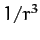is projected from infinity with a velocity that would carry it to a distance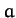from the center of force, if it were directed toward the latter. Actually, it is projected along a line whose closest distance from the center of force would beif there were no repulsion. Prove that the particle's least distance from the center is, and that the angle between the two asymptotes of its path is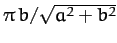.
2. A particle subject to a repulsive force varying as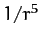is projected from infinity with a velocity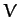that would carry it to a distancefrom the center of force, if it were directed toward the latter. Actually, it is projected along a line whose closest distance from the center of force would beif there were no repulsion. Show that the least velocity of the particle is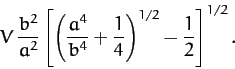3. Using the notation of Section 6.2, show that the angular momentum of a two-body system takes the formwhere.
4. Consider the case of Rutherford scattering in the event that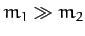. Demonstrate that the differential scattering cross-section in the laboratory frame is approximately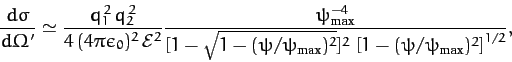where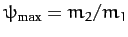.
5. Show that the energy distribution of particles recoiling from an elastic collision is always directly proportional to the differential scattering cross-section in the center of mass frame.
6. It is found experimentally that in the elastic scattering of neutrons by protons (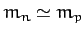) at relatively low energies the energy distribution of the recoiling protons in the laboratory frame is constant up to a maximum energy, which is the energy of the incident neutrons. What is the angular distribution of the scattering in the center of mass frame?
7. The most energetic-particles available to Earnst Rutherford and his colleagues for the famous Rutherford scattering experiment wereMeV. For the scattering of 7.7MeV-particles from Uranium 238 nuclei (initially at rest) at a scattering angle in the laboratory frame of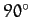, find the following (in the laboratory frame, unless otherwise specified):
1. The recoil scattering angle of the Uranium nucleus.
2. The scattering angles of the-particle and Uranium nucleus in the center of mass frame.
3. The kinetic energies of the scattered-particle and Uranium nucleus (in MeV).
4. The impact parameter,.
5. The distance of closest approach.
6. The differential scattering cross-section at.
8. Consider scattering by the repulsive potential(where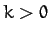) viewed in the center of mass frame. Demonstrate that the differential scattering cross-section is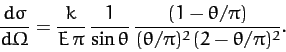Next: Rotating Reference Frames Up: Two-Body Dynamics Previous: Scattering in the Laboratory
Richard Fitzpatrick 2011-03-31